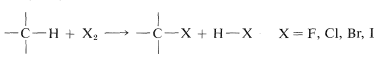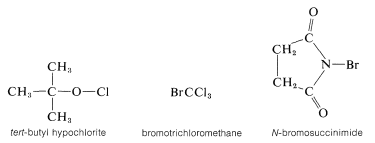# 4.6: Practical Halogenations and Problems of Selectivity

$$\newcommand{\vecs}{\overset { \rightharpoonup} {\mathbf{#1}} }$$ $$\newcommand{\vecd}{\overset{-\!-\!\rightharpoonup}{\vphantom{a}\smash {#1}}}$$$$\newcommand{\id}{\mathrm{id}}$$ $$\newcommand{\Span}{\mathrm{span}}$$ $$\newcommand{\kernel}{\mathrm{null}\,}$$ $$\newcommand{\range}{\mathrm{range}\,}$$ $$\newcommand{\RealPart}{\mathrm{Re}}$$ $$\newcommand{\ImaginaryPart}{\mathrm{Im}}$$ $$\newcommand{\Argument}{\mathrm{Arg}}$$ $$\newcommand{\norm}{\| #1 \|}$$ $$\newcommand{\inner}{\langle #1, #2 \rangle}$$ $$\newcommand{\Span}{\mathrm{span}}$$ $$\newcommand{\id}{\mathrm{id}}$$ $$\newcommand{\Span}{\mathrm{span}}$$ $$\newcommand{\kernel}{\mathrm{null}\,}$$ $$\newcommand{\range}{\mathrm{range}\,}$$ $$\newcommand{\RealPart}{\mathrm{Re}}$$ $$\newcommand{\ImaginaryPart}{\mathrm{Im}}$$ $$\newcommand{\Argument}{\mathrm{Arg}}$$ $$\newcommand{\norm}{\| #1 \|}$$ $$\newcommand{\inner}{\langle #1, #2 \rangle}$$ $$\newcommand{\Span}{\mathrm{span}}$$

Given the knowledge that a particular reaction will proceed at a suitable rate, a host of practical considerations are necessary for satisfactory operation. These considerations include interference by possible side reactions that give products other than those desired, the ease of separation of the desired product from the reaction mixture, and costs of materials, apparatus, and labor. We shall consider these problems in connection with the important synthetic reactions discussed in this book.

The chlorination of saturated hydrocarbons can be induced by light, but also can be carried out at temperatures of about $$300^\text{o}$$ in the dark. Under such circumstances the mechanism is similar to that of light-induced chlorination, except that the chlorine atoms are formed by thermal dissociation of chlorine molecules. Solid carbon surfaces catalyze thermal chlorination, possibly by aiding in the cleavage of the chlorine molecules.

Direct monohalogenation of saturated hydrocarbons works satisfactorily only with chlorine and bromine. For the general reactionthe calculated $$\Delta H^\text{0}$$ value is negative and very large for fluorine, negative and moderate for chlorine and bromine, and positive for iodine (see Table 4-7). With fluorine, the reaction evolves so much heat that it may be difficult to control, and products from cleavage of carbon-carbon as well as of carbon-hydrogen bonds may be obtained. The only successful, direct fluorination procedure for hydrocarbons involves diffusion of minute amounts of fluorine mixed with helium into liquid or solid hydrocarbons at low temperatures, typically $$-78^\text{o}$$ (Dry Ice temperature). As fluorination proceeds, the concentration of fluorine can be increased. The process is best suited for preparation of completely fluorinated compounds, and it has been possible to obtain in this way amounts of $$\left( CF_3 \right)_4C$$ and $$\left( CF_3 \right)_3 C-C \left( CF_3 \right)_3$$ from 2,2-dimethylpropane and 2,2,3,3-tetramethylbutane corresponding to $$10$$-$$15\%$$ yields based on the fluorine used.

Bromine generally is much less reactive toward hydrocarbons than chlorine is, both at high temperatures and with activation by light. Nonetheless, it usually is possible to brominate saturated hydrocarbons successfully. Iodine is unreactive.

Table 4-7: Calculated Heat of Reaction for Halogenation fo Hydrocarbons
Halogen (X) $$\Delta H^o$$ (kcal/mole)a
F -116
Cl -27
Br -10
I 13
aCalculated from the bond energies of Table 4-3.

The chlorination of methane does not have to stop with the formation of chloromethane (methyl chloride). It is usual when chlorinating methane to obtain some of the higher chlorination products: dichloromethane (methylene chloride), trichloromethane (chloroform), and tetrachloromethane (carbon tetrachloride):In practice, one can control the degree of substitution to a considerable extent by controlling the methane-chlorine ratio. For example, for monochlorination to predominate, a high methane-chlorine ratio is necessary such that the chlorine atoms react with $$CH_4$$ and not with $$CH_3Cl$$.

## Selectivity in Alkane Halogenation

For propane and higher hydrocarbons for which more than one monosubstitution product is generally possible, difficult separation problems bay arise when a particular product is desired. For example, the chlorination of 2-methylbutane $$3$$ at $$300^\text{o}$$ gives all four possible monosubstitution products, $$4$$, $$5$$, $$6$$, and $$7$$:On a purely statistical basis, we may expect the ratio of products from $$3$$ to correlate with the number of available hydrogens at the various positions of substitution. That is, $$4$$, $$5$$, $$6$$, and $$7$$ would be formed in the ratio 6:3:2:1 ($$50\%$$:$$25\%$$:$$17\%$$:$$8\%$$). However, as can be seen from Table 4-6, the strengths of hydrogen bonds to primary, secondary, and tertiary carbons are not the same and, from the argument given in Section 4-4E we would expect the weaker $$C-H$$ bonds to be preferentially attacked by $$Cl \cdot$$. The proportion of $$7$$ formed is about three times that expected on a statistical basis which is in accord with our expectation that the tertiary $$C-H$$ bond of 2-methylbutane should be the weakest of the $$C-H$$ bonds. (See Table 4-6.)

The factors governing selectivity in halogenation of alkanes follow:

1. The rates at which the various $$C-H$$ bonds of 2-methylbutane are broken by attack of chlorine atoms approach 1:1:1 as the temperature is raised above $$300^\text{o}$$. At higher temperatures both chlorine atoms and hydrocarbons become more reactive because of increases in their thermal energies. Ultimately, temperatures are attained where a chlorine atom essentially removes the first hydrogen with which it collides regardless of position on the hydrocarbon chain. In such circumstances, the composition of monochlorination products will correspond to that expected from simple statistics.

2. Bromine atoms are far more selective than chlorine atoms. This is not unexpected becauseis endothermic, whereas corresponding reactions with a chlorine atoms usually are exothermic (data from Table 4-6). Bromine removes only those hydrogens that are relatively weakly bonded to a carbon atom. As predicted, attack of $$Br \cdot$$ on 2-methylbutane leads mostly to 2-bromo-2-methylbutane, some secondary bromide, and essentially no primary bromides:3. The selectivity of chlorination reactions carried on in solution is increased markedly in the presence of benzene or alkyl-substituted benzenes because benzene and other arenes form loose complexes with chlorine atoms. This substantially cuts down chlorine-atom reactivity, thereby making the chlorine atoms behave more like bromine atoms.

## Chemical Initiation of Radical-Chain Substitution

It is possible to achieve chlorination of alkanes using sulfuryl chloride ($$SO_2Cl_2$$, bp $$69^\text{o}$$) in place of chlorine:The reaction has a radical-chain mechanism and the chains can be initiated by light or by chemicals, usually peroxides, $$ROOR$$. Chemical initiation requires an initiator with a weak bond that dissociates at temperatures between $$40$$-$$80^\text{o}$$. Peroxides are good examples. The $$O-O$$ bond is very weak ($$30$$-$$50 \: \text{kcal}$$) and on heating dissociates to alkoxyl radicals, $$RO \cdot$$, which are reactive enough to generate the chain-propagating radicals from the reactants. The exact sequence of chemical initiation is not always known, but a plausible route in the present case would have $$RO \cdot$$ abstract hydrogen from the alkane:The propagation steps that would follow areChlorination with sulfuryl chloride of alkanes with more than one kind of hydrogen gives a mixture of alkyl chlorides resembling that obtained with chlorine itself. However, in some circumstances the mixture of chlorides is not the same mixture obtained with chlorine itself and when this is true, the hydrogen-abstraction step probably involves $$\cdot SO_2Cl$$ rather than $$Cl \cdot$$. The alternative propagation steps then areDifferent product ratios are expected from $$Cl \cdot$$ and $$ClSO_2 \cdot$$ for the same reason that $$Cl \cdot$$ and $$Br \cdot$$ lead to different product ratios (Section 4-5A). Other reagents that sometimes are useful halogenating agents in radical-chain reactions include